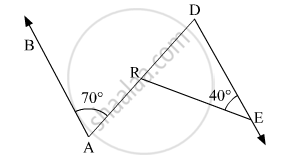Advertisement Remove all ads

# In the Given Figure, Line Ab || Line De. Find the Measures of ∠ Dre and ∠ Are Using Given Measures of Some Angles. - Geometry

Sum

In the given figure, line AB || line DE. Find the measures of ∠ DRE and ∠ ARE using given measures of some angles.Advertisement Remove all ads

#### Solution

AB || DE and AD is a transversal line.
∠BAR = ∠RDE = 70      (Alternate angles)
In △DER
∠D + ∠E + ∠DRE = 180   (Angle sum property)
⇒ 70 + 40 + ∠DRE = 180
⇒ 110 + ∠DRE = 180
⇒ ∠DRE = 70
Now, ∠ARE = ∠DRE + ∠RDE   (Exterior angle property)
= 70 + 40
= 110
Hence, the measures of ∠DRE and ∠ARE are 70∘ and 110respectively.

Is there an error in this question or solution?
Advertisement Remove all ads

#### APPEARS IN

Balbharati Mathematics 2 Geometry 9th Standard Maharashtra State Board
Chapter 3 Triangles
Practice Set 3.1 | Q 6 | Page 28
Advertisement Remove all ads
Advertisement Remove all ads
Share
Notifications

View all notifications

Forgot password?